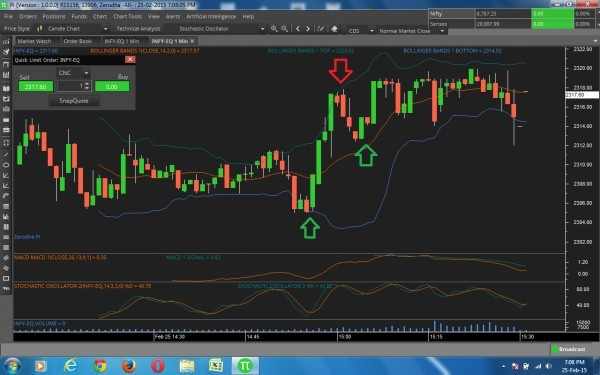# Code the following strategydear nitin

please make above strategy for buy and sell signal at stochestic and macd crossover with bolinger band support.

```SET A = SOPK(9, 3, 9, SIMPLE)
SET B = SOPD(9, 3, 9, SIMPLE)
SET C = MACD(13, 26, 9, SIMPLE
SET D = MACDSignal(13, 26, 9, SIMPLE)
CROSSOVER(A,B) AND CROSSOVER(C,D) AND
CLOSE>BBB(CLOSE, 20, 2, EXPONENTIAL)
```

Sell:

```SET A = SOPK(9, 3, 9, SIMPLE)
SET B = SOPD(9, 3, 9, SIMPLE)
SET C = MACD(13, 26, 9, SIMPLE
SET D = MACDSignal(13, 26, 9, SIMPLE)
CROSSOVER(B,A) AND CROSSOVER(D,C) AND
CLOSE<BBT(CLOSE, 20, 2, EXPONENTIAL)
```